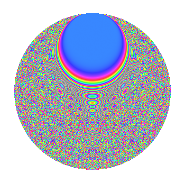# Properties

 Label 1512.2.diLevel 1512 Weight 2 Character orbit di Rep. character $$\chi_{1512}(115,\cdot)$$ Character field $$\Q(\zeta_{18})$$ Dimension 1704 Sturm bound 576

# Related objects

## Defining parameters

 Level: $$N$$ = $$1512 = 2^{3} \cdot 3^{3} \cdot 7$$ Weight: $$k$$ = $$2$$ Character orbit: $$[\chi]$$ = 1512.di (of order $$18$$ and degree $$6$$) Character conductor: $$\operatorname{cond}(\chi)$$ = $$1512$$ Character field: $$\Q(\zeta_{18})$$ Sturm bound: $$576$$

## Dimensions

The following table gives the dimensions of various subspaces of $$M_{2}(1512, [\chi])$$.

Total New Old
Modular forms 1752 1752 0
Cusp forms 1704 1704 0
Eisenstein series 48 48 0

## Trace form

 $$1704q - 3q^{2} - 18q^{3} - 3q^{4} - 6q^{8} - 6q^{9} + O(q^{10})$$ $$1704q - 3q^{2} - 18q^{3} - 3q^{4} - 6q^{8} - 6q^{9} - 6q^{11} - 9q^{12} + 9q^{14} - 3q^{16} - 3q^{18} - 12q^{22} - 9q^{24} - 6q^{25} - 18q^{26} - 12q^{28} + 33q^{30} - 33q^{32} - 18q^{33} + 18q^{34} - 6q^{35} - 42q^{36} + 81q^{38} - 54q^{40} + 9q^{42} - 24q^{43} + 3q^{44} + 3q^{46} - 12q^{49} - 27q^{50} + 66q^{51} - 9q^{52} - 135q^{54} - 69q^{56} - 6q^{57} - 3q^{58} - 18q^{59} - 33q^{60} - 99q^{62} - 6q^{64} - 6q^{65} - 9q^{66} - 6q^{67} + 36q^{68} - 60q^{70} - 111q^{72} - 18q^{73} + 69q^{74} - 18q^{75} + 36q^{76} - 30q^{78} - 18q^{80} - 6q^{81} - 18q^{82} + 180q^{83} + 66q^{84} - 81q^{86} + 45q^{88} + 81q^{90} - 6q^{91} - 72q^{92} - 9q^{94} - 9q^{96} + 111q^{98} - 24q^{99} + O(q^{100})$$

## Decomposition of $$S_{2}^{\mathrm{new}}(1512, [\chi])$$ into newform subspaces

The newforms in this space have not yet been added to the LMFDB.

## Hecke Characteristic Polynomials

There are no characteristic polynomials of Hecke operators in the database